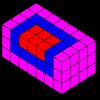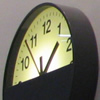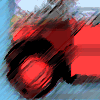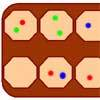# Resources tagged with: Visualising

Filter by: Content type:
Age range:
Challenge level:

### There are 178 results

Broad Topics > Mathematical Thinking > Visualising### Cuboid Challenge

##### Age 11 to 16 Challenge Level:

What's the largest volume of box you can make from a square of paper?### Sliced

##### Age 14 to 16 Challenge Level:

An irregular tetrahedron has two opposite sides the same length a and the line joining their midpoints is perpendicular to these two edges and is of length b. What is the volume of the tetrahedron?### Rati-o

##### Age 11 to 14 Challenge Level:

Points P, Q, R and S each divide the sides AB, BC, CD and DA respectively in the ratio of 2 : 1. Join the points. What is the area of the parallelogram PQRS in relation to the original rectangle?### Convex Polygons

##### Age 11 to 14 Challenge Level:

Show that among the interior angles of a convex polygon there cannot be more than three acute angles.### Clocked

##### Age 11 to 14 Challenge Level:

Is it possible to rearrange the numbers 1,2......12 around a clock face in such a way that every two numbers in adjacent positions differ by any of 3, 4 or 5 hours?### Königsberg

##### Age 11 to 14 Challenge Level:

Can you cross each of the seven bridges that join the north and south of the river to the two islands, once and once only, without retracing your steps?### Hidden Rectangles

##### Age 11 to 14 Challenge Level:

Rectangles are considered different if they vary in size or have different locations. How many different rectangles can be drawn on a chessboard?### Summing Squares

##### Age 14 to 16 Challenge Level:

Discover a way to sum square numbers by building cuboids from small cubes. Can you picture how the sequence will grow?### Tilting Triangles

##### Age 14 to 16 Challenge Level:

A right-angled isosceles triangle is rotated about the centre point of a square. What can you say about the area of the part of the square covered by the triangle as it rotates?### Hypotenuse Lattice Points

##### Age 14 to 16 Challenge Level:

The triangle OMN has vertices on the axes with whole number co-ordinates. How many points with whole number coordinates are there on the hypotenuse MN?### Pattern Power

##### Age 5 to 14

Mathematics is the study of patterns. Studying pattern is an opportunity to observe, hypothesise, experiment, discover and create.### Cubes Within Cubes

##### Age 7 to 14 Challenge Level:

We start with one yellow cube and build around it to make a 3x3x3 cube with red cubes. Then we build around that red cube with blue cubes and so on. How many cubes of each colour have we used?### Framed

##### Age 11 to 14 Challenge Level:

Seven small rectangular pictures have one inch wide frames. The frames are removed and the pictures are fitted together like a jigsaw to make a rectangle of length 12 inches. Find the dimensions of. . . .### Cubes Within Cubes Revisited

##### Age 11 to 14 Challenge Level:

Imagine starting with one yellow cube and covering it all over with a single layer of red cubes, and then covering that cube with a layer of blue cubes. How many red and blue cubes would you need?### Zooming in on the Squares

##### Age 7 to 14

Start with a large square, join the midpoints of its sides, you'll see four right angled triangles. Remove these triangles, a second square is left. Repeat the operation. What happens?### Speeding Boats

##### Age 14 to 16 Challenge Level:

Two boats travel up and down a lake. Can you picture where they will cross if you know how fast each boat is travelling?### Paving Paths

##### Age 11 to 14 Challenge Level:

How many different ways can I lay 10 paving slabs, each 2 foot by 1 foot, to make a path 2 foot wide and 10 foot long from my back door into my garden, without cutting any of the paving slabs?### Mystic Rose

##### Age 14 to 16 Challenge Level:

Use the animation to help you work out how many lines are needed to draw mystic roses of different sizes.### All in the Mind

##### Age 11 to 14 Challenge Level:

Imagine you are suspending a cube from one vertex and allowing it to hang freely. What shape does the surface of the water make around the cube?### Jam

##### Age 14 to 16 Challenge Level:

To avoid losing think of another very well known game where the patterns of play are similar.### How Many Dice?

##### Age 11 to 14 Challenge Level:

A standard die has the numbers 1, 2 and 3 are opposite 6, 5 and 4 respectively so that opposite faces add to 7? If you make standard dice by writing 1, 2, 3, 4, 5, 6 on blank cubes you will find. . . .### Christmas Chocolates

##### Age 11 to 14 Challenge Level:

How could Penny, Tom and Matthew work out how many chocolates there are in different sized boxes?### Partly Painted Cube

##### Age 14 to 16 Challenge Level:

Jo made a cube from some smaller cubes, painted some of the faces of the large cube, and then took it apart again. 45 small cubes had no paint on them at all. How many small cubes did Jo use?### AMGM

##### Age 14 to 16 Challenge Level:

Can you use the diagram to prove the AM-GM inequality?### Clocking Off

##### Age 7 to 16 Challenge Level:

I found these clocks in the Arts Centre at the University of Warwick intriguing - do they really need four clocks and what times would be ambiguous with only two or three of them?### Eight Hidden Squares

##### Age 7 to 14 Challenge Level:

On the graph there are 28 marked points. These points all mark the vertices (corners) of eight hidden squares. Can you find the eight hidden squares?### Square It

##### Age 11 to 16 Challenge Level:

Players take it in turns to choose a dot on the grid. The winner is the first to have four dots that can be joined to form a square.### Building Gnomons

##### Age 14 to 16 Challenge Level:

Build gnomons that are related to the Fibonacci sequence and try to explain why this is possible.### Triangles Within Pentagons

##### Age 14 to 16 Challenge Level:

Show that all pentagonal numbers are one third of a triangular number.### Triangles Within Squares

##### Age 14 to 16 Challenge Level:

Can you find a rule which relates triangular numbers to square numbers?### Triangles Within Triangles

##### Age 14 to 16 Challenge Level:

Can you find a rule which connects consecutive triangular numbers?### Buses

##### Age 11 to 14 Challenge Level:

A bus route has a total duration of 40 minutes. Every 10 minutes, two buses set out, one from each end. How many buses will one bus meet on its way from one end to the other end?### Something in Common

##### Age 14 to 16 Challenge Level:

A square of area 3 square units cannot be drawn on a 2D grid so that each of its vertices have integer coordinates, but can it be drawn on a 3D grid? Investigate squares that can be drawn.### Bands and Bridges: Bringing Topology Back

##### Age 7 to 14

Lyndon Baker describes how the Mobius strip and Euler's law can introduce pupils to the idea of topology.### Coordinate Patterns

##### Age 11 to 14 Challenge Level:

Charlie and Alison have been drawing patterns on coordinate grids. Can you picture where the patterns lead?##### Age 11 to 14 Challenge Level:

Can you mark 4 points on a flat surface so that there are only two different distances between them?### Sliding Puzzle

##### Age 11 to 16 Challenge Level:

The aim of the game is to slide the green square from the top right hand corner to the bottom left hand corner in the least number of moves.### Concrete Wheel

##### Age 11 to 14 Challenge Level:

A huge wheel is rolling past your window. What do you see?### Changing Places

##### Age 14 to 16 Challenge Level:

Place a red counter in the top left corner of a 4x4 array, which is covered by 14 other smaller counters, leaving a gap in the bottom right hand corner (HOME). What is the smallest number of moves. . . .### Inside Out

##### Age 14 to 16 Challenge Level:

There are 27 small cubes in a 3 x 3 x 3 cube, 54 faces being visible at any one time. Is it possible to reorganise these cubes so that by dipping the large cube into a pot of paint three times you. . . .### Dice, Routes and Pathways

##### Age 5 to 14

This article for teachers discusses examples of problems in which there is no obvious method but in which children can be encouraged to think deeply about the context and extend their ability to. . . .### On the Edge

##### Age 11 to 14 Challenge Level:

If you move the tiles around, can you make squares with different coloured edges?### Wari

##### Age 14 to 16 Challenge Level:

This is a simple version of an ancient game played all over the world. It is also called Mancala. What tactics will increase your chances of winning?### Jam

##### Age 14 to 16 Challenge Level:

A game for 2 players### Frogs

##### Age 11 to 14 Challenge Level:

How many moves does it take to swap over some red and blue frogs? Do you have a method?### Painted Cube

##### Age 14 to 16 Challenge Level:

Imagine a large cube made from small red cubes being dropped into a pot of yellow paint. How many of the small cubes will have yellow paint on their faces?### Picturing Square Numbers

##### Age 11 to 14 Challenge Level:

Square numbers can be represented as the sum of consecutive odd numbers. What is the sum of 1 + 3 + ..... + 149 + 151 + 153?### Picturing Triangular Numbers

##### Age 11 to 14 Challenge Level:

Triangular numbers can be represented by a triangular array of squares. What do you notice about the sum of identical triangle numbers?### Fermat's Poser

##### Age 14 to 16 Challenge Level:

Find the point whose sum of distances from the vertices (corners) of a given triangle is a minimum.### Coloured Edges

##### Age 11 to 14 Challenge Level:

The whole set of tiles is used to make a square. This has a green and blue border. There are no green or blue tiles anywhere in the square except on this border. How many tiles are there in the set?